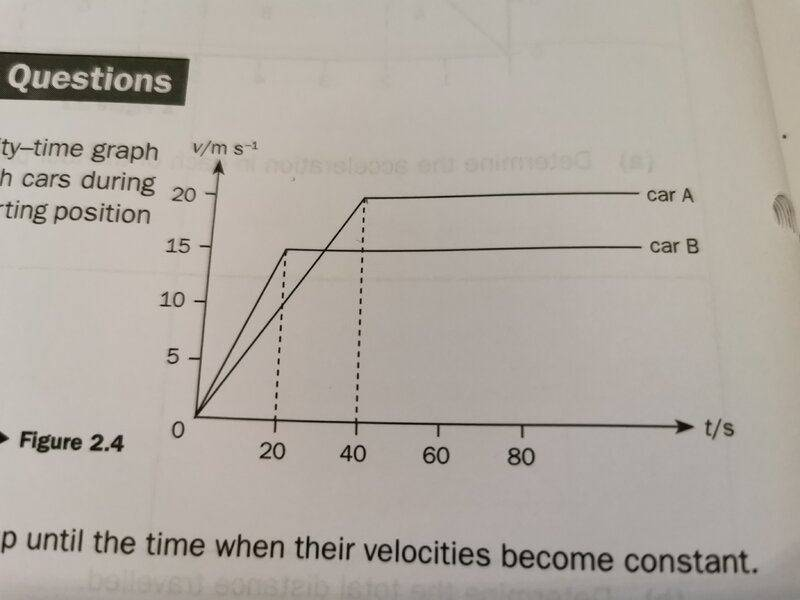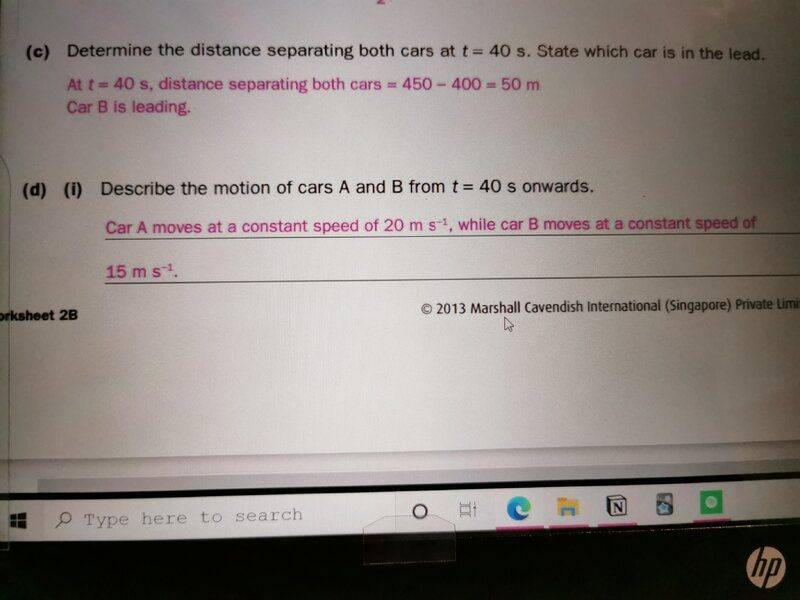# Can you use speed and velocity interchangeably?

ellieee
Homework Statement:
qn (di)-> the graph is a v-t graph, so shouldn't we say "constant velocity" instead of "speed"?
Relevant Equations:
nilMentor
Yes. It's better to describe it as constant velocity, as speed just describes the magnitude and not the sign. (I presume this is one-dimensional motion.)

Homework Helper
Gold Member
In motion in a straight line such as in your example, the concepts of velocity and speed are almost identical: If speed is x, then velocity will be x or -x. I repeat, this holds only in motion in a straight line, it does not hold for other motions e.g. circular motion.

ellieee
in a straight line
how can you tell it's in a straight line ?

Last edited by a moderator:
Homework Helper
Gold Member
how can u tell it's in a straight line ?
I can not infer it from the given data (the screenshot of the graph isn't complete, doesn't completely show the text that describes the graph), somehow I thought it was given to you.

Mentor
how can u tell it's in a straight line ?
Since they label it a velocity-time graph, where the velocity is constant it must be moving in a straight line. (Assuming it's labeled accurately.)